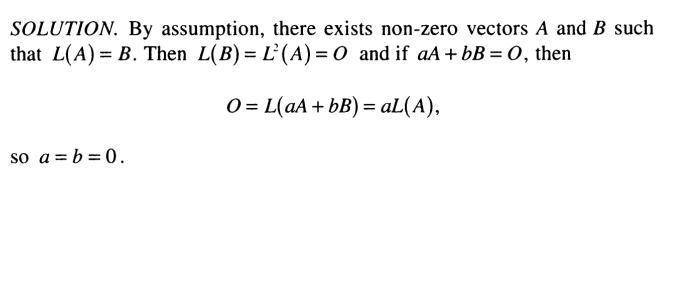Linear algebra-Basis of a linear map

Homework Statement

Let ##L: R^{2} → R^{2}## be a linear map such that ##L ≠ O## but## L^{2} = L \circ L = O.##
Show that there exists a basis {##A##, ##B##} of ##R^{2}## such that:

##L(A) = B## and ##L(B) = O.##​

The Attempt at a Solution

Here's the solution my book provides :Well I have two questions:
1.Why do they say that ##aA+bB=O##???. I mean I don't understand the solution from that point until the end (Why the solutions ##a=0## and ##b=0## are enough to prove the existence of that basis??May someone please explain??
Thanks in advance. Any help would be appreciated

The solution says IF $aA+bB=0$, THEN $a=b=0$. That is what it means for the vectors $A$ and $B$ to be linearly independent. Vectors in a basis must be linearly independent.

The solution says IF $aA+bB=0$, THEN $a=b=0$. That is what it means for the vectors $A$ and $B$ to be linearly independent. Vectors in a basis must be linearly independent.
Thanks but why ##O=L(aA+bB)=aL(A)##?? could you please explain what they do there, please??

Thanks but why ##O=L(aA+bB)=aL(A)##?? could you please explain what they do there, please??

$L$ is a linear map, which means $L(aA+bB) = aL(A) + bL(B)$.

•1 person
$L$ is a linear map, which means $L(aA+bB) = aL(A) + bL(B)$.

Thanks